# Constructing the Tangent at a Point on a Circle

Compass and straight edge constructions are of interest to mathematicians, not only in the field of geometry, but also in algebra. For thousands of years, beginning with the Ancient Babylonians, mathematicians were interested in the problem of "squaring the circle" (drawing a square with the same area as a circle) using a straight edge and compass. This problem is equivalent to finding the area of a circle. It turns out that this is impossible, but no-one managed to prove this until 1882!

However, it is possible to construct the tangent at a point on a circle using only a straight edge and compass. For this construction, you will need a straight edge (ruler - but you won't be measuring anything), a pair of compasses, a pencil and paper. I have drawn the pictures using the robocompass app. It's fun to play with, and you can use it to do all sorts of geometric constructions. There's a little bit of coding to learn, but a list of instructions is provided. Once you've written your little program, you can invite a few friends over to watch the construction. Be warned that, when you are using it to draw arcs, the robocompass compass point may appear to be a little away from the centre, but the drawing is actually accurate.

## The Construction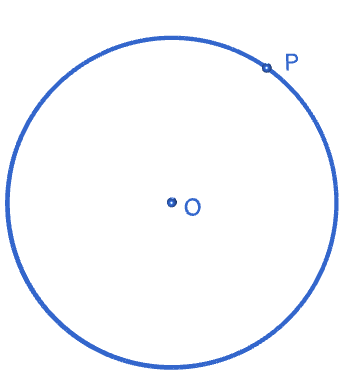Step 1: Start with the circle, with its centre $O$ and the point $P$ that you want your tangent to pass through. If you don't know where the centre of your circle is, don't guess! There's a construction that you can use to find it. Read all about it in the article on constructing the centre of a circle.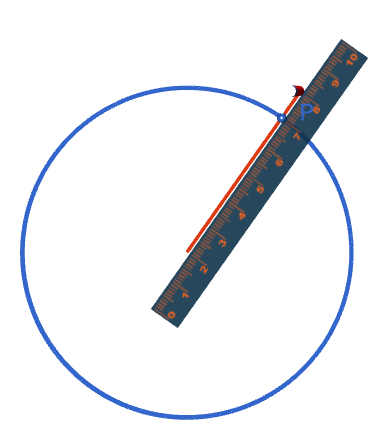Step 2: Use your straight edge to draw a line segment joining the centre $O$ of the circle and the point $P$. Make sure it extends a fair way past point $P$. The picture on the right shows what this should look like when you're finished.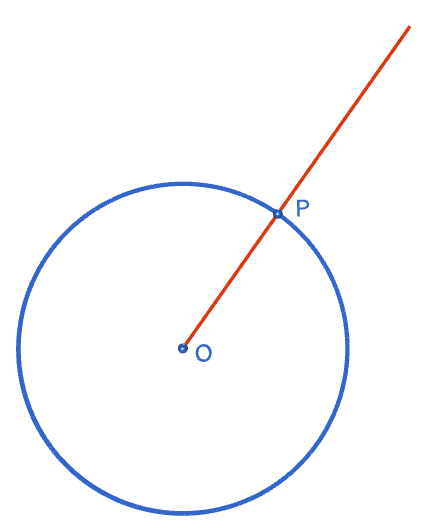Step 3: Now we're going to construct a perpendicular to the straight line $OP$ at the point $P$. The steps are the same as the steps for constructing a perpendicular to a line at a point. Place the tip of the compasses at point $P$ and open them to a radius that is less than the distance from point $O$ to point $P$. Draw an arc on each side of the point $P$ that cuts the line through $O$ and $P$. Label the points where the arcs cut the line segment with $Q$ and $R$. The picture on the right shows what your construction should look like when you've completed this step.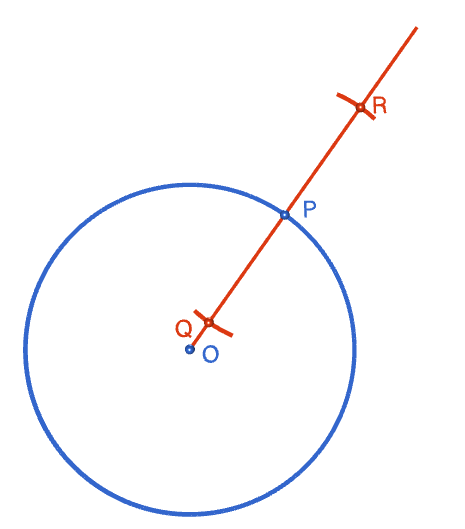Step 4: Open your compasses to any radius greater than the distance from $Q$ to $P$. With the tip of the compasses at point $Q$, draw an arc on one side of the point $P$, in a similar position to the one shown in the diagram on the left.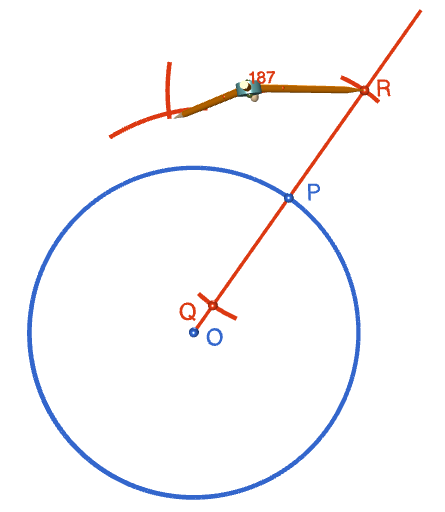Step 5: Keeping the same radius, move the tip of the compasses to point $R$ and draw a second arc, intersecting the arc drawn in step 4.Step 6: Use your straight edge to draw a line segment passing through point $P$ and the point where the two arcs from steps 4 and 5 intersect. This is your tangent to the circle. The picture on the right shows the completed construction.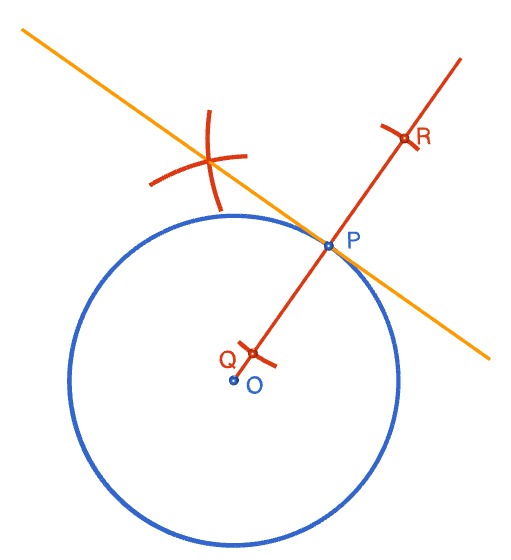### Description

This tutorial will expose you to Rulers and how to use them. We will also have a close look as set square and compass constructions. Your feedback is important to us, if you like any other topic covered under this tutorial, please do let us know.

### Audience

Year 10 or higher

### Learning Objectives

Ruler and compass constructions and more

Author: Subject Coach
You must be logged in as Student to ask a Question.